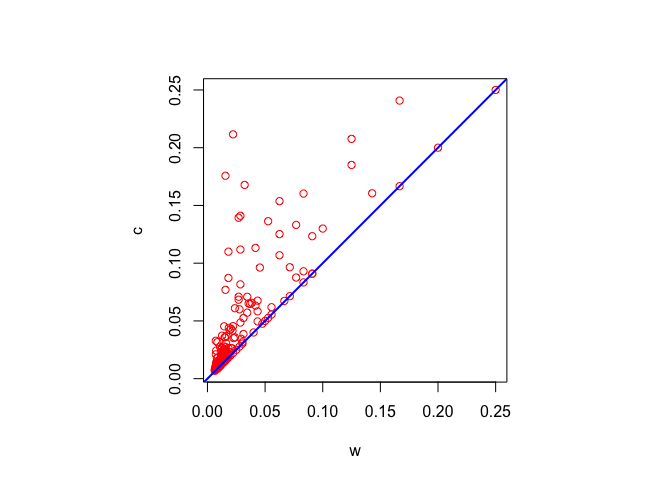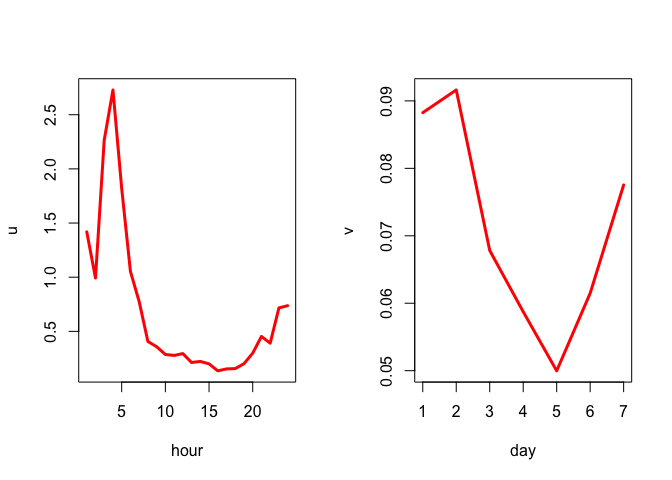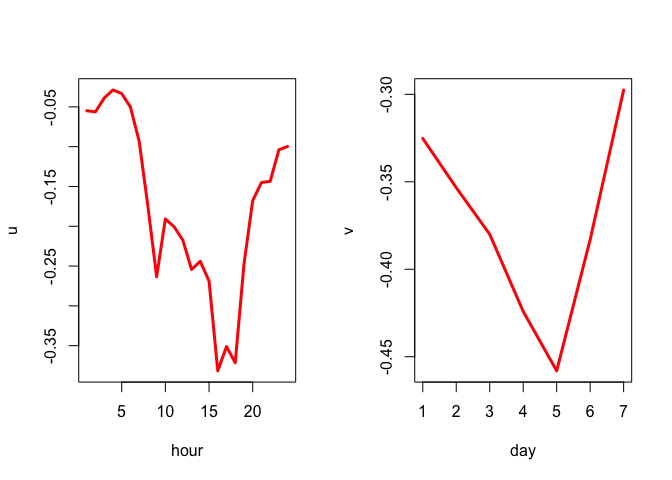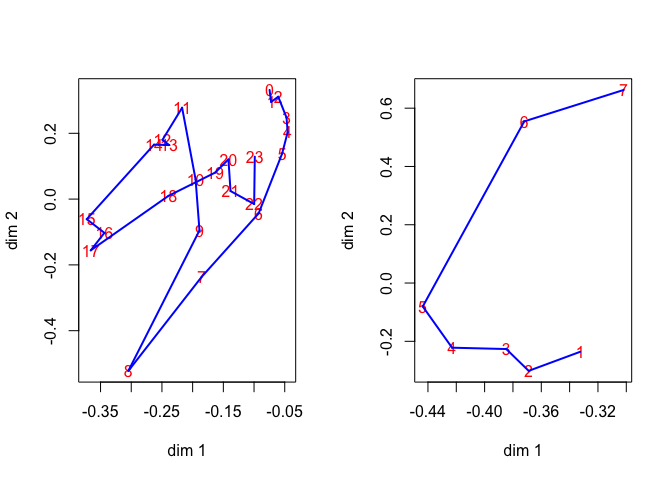Note: This is a working paper which will be expanded/updated frequently. All suggestions for improvement are welcome. The directory deleeuwpdx.net/pubfolders/linlow has a pdf version, the complete Rmd file with all code chunks, the R code, and the bib file.

# 1 Introduction

In a rank-p matrix approximation problem we have an $$n\times m$$ matrix $$X$$ and we want to find the closest $$n\times m$$ matrix $$\widehat{X}$$ of rank-p in the least squares sense (Eckart and Young (1936), Keller (1962)). The problem is usually parametrized as $$\widehat X=AB'$$ where $$A$$ is $$n\times p$$ and $$B$$ is $$m\times p$$. If closeness is measured by the usual Frobenius norm, i.e. the unweighted sum of squares, then the solution is given by the first $$p$$ singular values of $$X$$ and their corresponding left and right singular vectors. Generalizations to more general Euclidean norms are discussed, with some historical references, in De Leeuw (1984) (and undoubtedly in hundreds of other places). In this paper we generalized to simple weighted least squares in which the square of each residual $$x_{ij}-\widehat x_{ij}$$ is weighted by a non-negative weight $$w_{ij}$$. Thus the loss function we must minimize is $\sigma(A,B):=\sum_{i=1}^n\sum_{j=1}^mw_{ij}(x_{ij}-\sum_{s=1}^pa_{is}b_{js})^2.$ This general problem was perhaps first tackled by Gabriel and Zamir (1979), using an alternating least squares algorithm of the form (with superscript $$k$$ indicating iteration count) \begin{align*} A^{(k)}&=\mathop{\mathbf{argmin}}_A\ \sigma(A,B^{(k)}),\\ B^{(k+1)}&=\mathop{\mathbf{argmin}}_B\ \sigma(A^{(k)},B). \end{align*}

Both subproblems of this criss-cross regression algorithm are weighted linear least squares problems, with a possibly non-sparse matrix of weights. We will go a different route, by concentrating on simplifying the weights.

# 2 Majorization using Weights

Majorization algorithms are discussed in general in De Leeuw (1994) and Heiser (1995), and more recently and more extensively in Lange (2016) and De Leeuw (2016a). We assume the basic majorization approach is familiar and go straight away to the details.

Our first job is to construct a majorization scheme.

Suppose $$A^{(k)}$$ and $$B^{(k)}$$ are solutions after $$k$$ iterations. Define $\hat x_{ij}^{(k)}:=\sum_{s=1}^pa_{is}^{(k)}b_{js}^{(k)}.$ Then $\sum_{s=1}^pa_{is}b_{js}=\hat x_{ij}^{(k)}+(\sum_{s=1}^pa_{is}b_{js}-\hat x_{ij}^{(k)}),$ and consequently $\sigma(A,B)= \sigma(A^{(k)},B^{(k)})-2\sum_{i=1}^n\sum_{j=1}^mw_{ij}(x_{ij}-\hat x_{ij}^{(k)})(\sum_{s=1}^pa_{is}b_{js}-\hat x_{ij}^{(k)})+ \sum_{i=1}^n\sum_{j=1}^mw_{ij}(\sum_{s=1}^pa_{is}b_{js}-\hat x_{ij}^{(k)})^2.$ If $$c_{ij}\geq w_{ij}$$ and $$c_{ij}>0$$ we have $\sigma(A,B)\leq\sigma(A^{(k)},B^{(k)})-2\sum_{i=1}^n\sum_{j=1}^mw_{ij}(x_{ij}-\hat x_{ij}^{(k)})(\sum_{s=1}^pa_{is}b_{js}-\hat x_{ij}^{(k)})+ \sum_{i=1}^n\sum_{j=1}^mc_{ij}(\sum_{s=1}^pa_{is}b_{js}-\hat x_{ij}^{(k)})^2$ Define $r_{ij}^{(k)}:=\frac{w_{ij}}{c_{ij}}(x_{ij}-\widehat x_{ij}^{(k)}),$ and $h_{ij}^{(k)}:=\widehat x_{ij}^{(k)}+\frac{w_{ij}}{c_{ij}}(x_{ij}-\hat x_{ij}^{(k)}).$ Note that $$h_{ij}^{(k)}$$ is a convex combination of $$\widehat x_{ij}^{(k)}$$ and $$x_{ij}$$. Now $\sigma(A,B)\leq\sigma(\alpha,\beta)-2\sum_{i=1}^n\sum_{j=1}^mc_{ij}\{r_{ij}^{(k)}\}^2+ \sum_{i=1}^n\sum_{j=1}^mc_{ij}(\sum_{s=1}^pa_{is}b_{js}-\widehat h_{ij}^{(k)})^2$ In the majorization step we minimize $\eta(A,B,A^{(k)},B^{(k)}):=\sum_{i=1}^n\sum_{j=1}^mc_{ij}(\sum_{s=1}^pa_{is}b_{js}-\widehat h_{ij}^{(k)})^2,$ which is a matrix approximation problem similar to our original problem, with adjusted weights and an adjusted target. In the next iteration we define a new target, solve the matrix approximation problem again, and so on.

It seems that our developments so far have not gained us much. Instead of one matrix approximation problem we now have a sequence of them, all with the same structure. The gain will have to come from the choice of the $$c_{ij}$$, which we will try to construct in such a way that the matrix approximation problems in each iteration become more simple.

## 2.1 Rank-one Weights

First suppose $$c_{ij}>0$$ and there are $$u>0$$ and $$v>0$$ such that $$c_{ij}=u_iv_j$$. Now $g_{ij}^{(k)}:=\sqrt{u_iv_j}\ \widehat h_{ij}^{(k)},$ and define new variables $$\alpha_{is}=\sqrt{u_i}a_{is}$$ and $$\beta_{js}=\sqrt{v_j}b_{js}$$. Then $\sum_{i=1}^n\sum_{j=1}^mc_{ij}(\sum_{s=1}^pa_{is}b_{js}-\widehat h_{ij}^{(k)})^2=\sum_{i=1}^n\sum_{j=1}^m(\sum_{s=1}^p\alpha_{is}\beta_{js}-g_{ij}^{(k)})^2$ This shows that the matrix approximation problems that must be solved in each majorization step are unweighted, which means they can be solved rapidly using partial singular value decomposition.

## 2.2 The Symmetric Case

If $$X$$ is symmetric our loss function becomes $\sigma(A)=\sum_{i=1}^n\sum_{j=1}^n w_{ij}(x_{ij}-\sum_{s=1}^p a_{is}a_{js})^2.$ Now choose $$c_{ij}=u_iu_j\leq w_{ij}$$, and basically the same majorization scheme can be applied. The matrix approximation problem in each iteration is now solved by using the first $$p$$ non-negative eigenvalues of the current target (Keller (1962), De Leeuw (1974)).

## 2.3 Some History

From an historical point of view the most important special case is that in which $$w_{ij}$$ is either one or zero, which zero indicating missing data. It was analyzed by Thomson (1934) in the case of factor analysis, using basically the same alternating least squares algorithm used by Yayes (1933) in factorial experiments.

We derive this algorithm from our majorization perspective by choosing $$c_{ij}=1$$, no matter if $$w_{ij}=1$$ or $$w_{ij}=0$$. Thus $r_{ij}^{(k)}=w_{ij}(x_{ij}-\hat x_{ij}^{(k)})=\begin{cases}0&\text{ if }w_{ij}=0,\\x_{ij}-\hat x_{ij}^{(k)}&\text{ if }w_{ij}=1,\end{cases}$ and $h_{ij}^{(k)}=\hat x_{ij}^{(k)}+r_{ij}^{(k)}=\begin{cases}\hat x_{ij}^{(k)}&\text{ if }w_{ij}=0,\\x_{ij}&\text{ if }w_{ij}=1.\end{cases}$ This is exactly the Thomson-Yates method for dealing with data that are missing, either by design, by accident, or structurally from properties of the model. The equivalence of the alternating least squares and majorization methods in this case was already observed by Kiers (1997).

Kiers (1997), following some suggestions by Heiser (1995), proposes to use $$c_{ij}=\max_{i=1}^n\max_{j=1}^m w_{ij}$$. Thus all elements of $$c_{ij}$$ are the same, say equal to $$c$$, and $h_{ij}^{(k)}=\hat x_{ij}^{(k)}+\frac{w_{ij}}{c}(x_{ij}-\hat x_{ij}^{(k)}).$ This is improved by Groenen, Giaquinto, and Kiers (2003) by using $$c_{ij}=\max_{j=1}^m w_{ij}$$, which means that we can write $h_{ij}^{(k)}=\hat x_{ij}^{(k)}+\frac{w_{ij}}{c_i}(x_{ij}-\hat x_{ij}^{(k)}).$ Both Kiers (1997) and Groenen, Giaquinto, and Kiers (2003) compare their majorization algorithms with the alternating least squares method of Gabriel and Zamir (1979). The conclusion seems to be that the algorithm of Kiers (1997), which uses a scalar bound for the $$w_{ij}$$ is slower than criss-cross regression, while the algorithm of Groenen, Giaquinto, and Kiers (2003), which uses a row-wise bound, is actually faster than criss-cross regression.

It is clear that both Kiers (1997) and Groenen, Giaquinto, and Kiers (2003) use $$c_{ij}$$ of the form $$c_{ij}=u_iv_j$$, with different choices of $$u$$ and $$v$$. We will now look at some general theory for weight majorizations of this form, and see if we can come up with possible improvements.

## 2.4 Rate of Convergence

The algorithmic map $$\mathcal{A}:\mathbb{R}^{n\times m}\rightarrow\mathbb{R}^{n\times m}$$ in the case that $$C=uv'$$ is defined as the composition of three maps.

• The first is the affine map $$\mathcal{G}(Z):=U^\frac12(Z+F\star(X-Z))V^\frac12,$$ where $$\star$$ is used for the Hadamard product, and $$F$$ has elements $$f_{ij}=w_{ij}/c_{ij}$$. The diagonal matrices $$U$$ and $$V$$ have the elements of $$u$$ and $$v$$ on the diagonal.
• The second map $$\Gamma_p$$ non-linearly projects its argument, using the Frobenius norm, on the set of all matrices of rank less than or equal to $$p$$.
• And finally we have the linear map $$\mathcal{S}(Z):=U^{-\frac12}ZV^{-\frac12}$$.

Combining these three maps, all from $$\mathbb{R}^{n\times m}$$ to $$\mathbb{R}^{n\times m}$$, $\mathcal{A}(Z)=\mathcal{S}(\Gamma_p(\mathcal{G}(Z))).$

The asymptotic error constant of the iterative majorization procedure is the spectral radius of the derivative of the algorithmic map $$\mathcal{A}$$. So we now proceed to give an expression for that derivative. By the chain rule $\mathcal{A}(Z+\Delta)=\mathcal{A}(Z)+\mathcal{S}(\mathcal{D}\Gamma_p(\mathcal{G}(Z))\mathcal{H}(\Delta))+\mathcal{o}(\|\Delta\|),$ where $$\mathcal{H}(\Delta)=U^\frac12(E\star\Delta)V^\frac12$$ and $$E$$ is the matrix with elements $$1-w_{ij}/c_{ij}$$.

The derivatives of $$\Gamma_p$$ in the rectangular case are computed in De Leeuw (2008) (the symmetric case is in De Leeuw (2016b)). From De Leeuw (2008), using the representation $$\Gamma_p(\mathcal{G}(Z))=\mathcal{G}(Z)L_p^{\ }L_p'$$, with $$L_p$$ the normalized eigenvectors corresponding with the $$p$$ largest eigenvalues of $$\mathcal{G}(Z)'\mathcal{G}(Z)$$, $\mathcal{D}\Gamma_p(\mathcal{G}(Z))\mathcal{H}(\Delta)=\mathcal{H}(\Delta)L_p^{}L_p'-\mathcal{G}(Z)\{\Xi_p^{}(Z,\Delta)+\Xi_p'(Z,\Delta)\},$ where $\Xi_p(Z,\Delta)=\sum_{s=1}^p (\mathcal{G}(Z)'\mathcal{G}(Z)-\lambda_s^2I)^+(\mathcal{G}(Z)'\mathcal{H}(\Delta)+\mathcal{H}(\Delta)'\mathcal{G}(Z))l_s^{}l_s'.$ This allows us to compute the partial derivatives by using unit matrices (one element one, the others zero) for $$\Delta$$. Directional derivatives for general $$\Delta$$ are implemented in lrPerturb() and the matrix of partials in computed in lrDerive(), both functions are in the file lowrank.R.

# 3 One-sided Approximation

As a consequence of our previous discussions it makes sense to look for $$u\geq 0$$ and $$v\geq 0$$ such that $$u_iv_j\geq w_{ij}$$ for all $$i,j$$, but, given the constraints, the matrix with elements $$u_iv_j$$ is as small as possible. There are many ways in which we can formalize “as small as possible”. In De Leeuw (2017) convex analysis and duality were used in a different and more general context. In this paper we use the notion of approximation from above, i.e. we minimize some norm $$\|W-uv'\|$$ over $$uv'\geq W$$ and $$u,v\geq 0$$.

In order to make this a convex problem we take logaritms. Thus we minimize $$\|\log W -(\log u+\log v)\|$$ over $$\log u_i + \log v_j\geq\log w_{ij}$$ for all $$i$$ and $$j$$. One-sided discrete linear approximation for general $$\ell_p$$ norms is discussed in Watson (1973). We will use least squares on the logarithms, which leads to a quadratic programming problem. But $$\ell_1$$ and $$\ell_\infty$$ are both interesting as well, and lead to linear programming problems.

Using logarithms only makes sense if $$w_{ij}>0$$. For $$w_{ij}=0$$ there is an obvious way to proceed, by just skipping those elements. The $$u_i$$ and $$v_j$$ computed over the non-zero $$w_{ij}$$ will automatically satisfy $$u_iv_j>w_{ij}=0$$ for the zero weights as well.

Suppose $$\mathcal{M}$$ is the set of pairs $$(i,j)$$ such that $$w_{ij}>0$$.To solve minimization of $\mathop{\sum\sum}_{(i,j)\in\mathcal{M}}\ (\log w_{ij}-\log u_i-\log v_j)^2$ over over $$\log u_i + \log v_j\geq\log w_{ij}$$ we use the \lsi() function from the lsei package (Wang, Lawson, and Hanson (2015)).

# 4 Example

The data are crash data on New Zealand roads, from the VGAM package (Yee (2010)). Data are cross-classified by time (hour of the day) and day of the week, accumulated over 2009. We use crashi, which gives the number of injuries by caused by cars.

##    Mon Tue Wed Thu Fri Sat Sun
## 0   16  10  22  12  29  55  55
## 1   13  11  15  23  23  42  64
## 2    5   8  16  13  24  37  64
## 3    6   4   8  12  19  31  45
## 4    7   6  11  16  11  35  35
## 5   12  14  14  18  19  27  35
## 6   37  37  32  45  32  21  36
## 7   66  79  92  75  73  40  33
## 8  117 138 132 138 122  59  36
## 9   67  81  68  75  72  59  45
## 10  67  70  62  76  72  84  57
## 11  80  80  50  80  74 114  86
## 12  75  85  86  87  94 101  93
## 13  77  69  84  90  90 105  80
## 14  84  87  98  85 104 103  96
## 15 112 136 134 156 158 120 103
## 16 115 110 138 144 146 106  90
## 17 127 130 140 149 155 104  97
## 18  63  69  91  97 142  83  64
## 19  47  63  53  57  67  69  52
## 20  25  46  62  55  68  70  44
## 21  34  42  49  53  85  62  33
## 22  24  26  35  52  67  54  18
## 23  28  23  20  49  61  69  29

We pretend the data are independent Poisson counts, and we choose weights equal to $$x_{ij}^{-1}$$. This makes the minimum of the loss function a chi-square random variable, with the appropriate number of degrees of freedom. The optimal rank-one approximation from above is computed wth the program mbound() from the appendix. The optimal $$uv'$$ is plotted against $$w$$ in figure 1 and the optimal $$u$$ and $$v$$ are plotted in figure 2.Figure 1: Best Rank-1 Approximation WeightsFigure 2: Components of One-dimensional Bounds

The wPCA() function has a bnd parameter, which can be equal to all, or col, or row, or opt. If bnd="all" then $$c_{ij}=\max w_{ij}$$, as in Kiers (1997), if bnd="row" then $$c_{ij}=\max_j w_{ij}$$, as in Groenen, Giaquinto, and Kiers (2003). If bnd="col" then $$c_{ij}=\max_i w_{ij}$$ and if bnd="opt" then we compute the optimal $$u_iv_j$$.

First look at $$p=1$$. For all four values of the bnd parameter we find a chi-square equal to 709.9526292976 with $$168-((24+7)-1)=138$$ degrees of freedom. The iteration count for bnd="all","col","row","opt" is, respectively, 208, 151, 21, 17. Because in this example the between-row/within-column variation is so much larger than the within-row/between-column variation, it makes sense that bnd="row" performs well, almost as good as bnd="opt", while bnd="col" is bad, almost as bad as bnd="all". The one-dimensional plots of $$A$$ and $$B$$ from $$\widehat X=ab'$$ are in figure 3.Figure 3: Components of One-dimensional Matrix Approximation

We also computed the spectral norms of the derivative at the solution, using the functions in lowrank.R. For bnd="all","col","row","opt" the convergence rate is, respectively, 0.9710924907, 0.955475149, 0.660381091, 0.6152936489.

For $$p=2$$ for all four values of the bnd parameter we find chi-square equal to 215.349822881 with $$168-((24+7)*2-4)=110$$ degrees of freedom. The iteration count for bnd="all","col","row","opt" is, respectively, 164, 99, 46, 35.Figure 4: Components of Two-dimensional Matrix Approximation

For bnd="all","col","row","opt" the convergence rate is, respectively, 0.9715807406, 0.961724624, 0.9042846128, 0.8856193743.

# 5 Discussion

The main question, which we did not answer, is if the decrease in computer time by decreasing the number of iterations is offset by the extra computation of the optimal rank-one bounds. Computing the optimal bounds is a quadratic programming problem of size $$nm$$, which can be substantial and rapidly becomes impractical for really large data sets. In that case, alternative strategies are needed, for example starting with row or column bounds and performing only a few iterations of an iterative quadratic programming method. Of course we also have not tried $$\ell_1$$ and $$\ell_\infty$$ loss functions to define the optimal weights, which have linear programming solutions that are less expensive than the quadratic ones.

Also note that in each majorization step we have solved a low-rank approximation problem. We also have a convergent algorithm if we replaced the singular value decomposition in each majorization step by one or more alternating least squares iterations. This will generally lead to more majorization iterations, but each majorization iteration becomes less expensive. In addition we can use $$A$$ and $$B$$ from $$\widehat X=AB'$$ for the previous majorization iteration as starting points for the next one.

There is an interesting recent paper by Szlam, Tulloch, and Tygert (2017), who show that just a few alternating least squares iterations will generally provide a very good low-rank approximation. This implies that it may not pay off to optimize the number of iterations needed for convergence, if convergence is not really of much practical value anyway. It also indicates that performing only a small number of “inner” alternating least squares iterations in each majorization step is probably a good strategy. Again, that is something to be explored.

# 6 Appendix: Code

## 6.1 mbound.R

mbound <- function (w) {
n <- nrow (w)
m <- ncol (w)
ww <- log (w)
g1 <- matrix(0, n * m, n)
g2 <- matrix(0, n * m, m)
k <- 1
for (j in 1:m) {
for (i in 1:n) {
g1[k, i] <- 1
g2[k, j] <- 1
k <- k + 1
}
}
g <- cbind (g1, g2)
f <- log (as.vector (w))
h <- lsi (g, f, g, f)
u <- exp (h[1:n])
v <- exp (h[n + (1:m)])
return (list (u = u, v = v))
}

## 6.2 wPCA.R

gfunc <- function (z, x, u, v, w) {
return ((z + (w / outer (u, v)) * (x - z)) * sqrt(outer(u,v)))
}

sfunc <- function (z, u, v) {
return (z / sqrt (outer (u, v)))
}

lfunc <- function (x, p) {
l <- as.matrix(eigen (crossprod(x))$vectors[,1:p]) return (x %*% tcrossprod (l)) } wPCA <- function (x, w, p = 2, bnd = "opt", itmax = 1000, eps = 1e-6, verbose = FALSE) { n <- nrow (x) m <- ncol (x) if (bnd == "all") { u <- rep(1, n) v <- rep (max (w), m) } if (bnd == "row") { u <- apply (w, 1, max) v <- rep (1, m) } if (bnd == "col") { u <- rep (1, n) v <- apply (w, 2, max) } if (bnd == "opt") { uv <- mbound (w) u <- uv$u
v <- uv$v } zold <- lfunc (x, p) fold <- sum (w * (x - zold) ^ 2) itel <- 1 repeat { z1 <- gfunc (zold, x, u, v, w) z2 <- lfunc (z1, p) znew <- sfunc (z2, u, v) fnew <- sum (w * (x - znew) ^ 2) if (verbose) { cat ( "iteration ", formatC ( itel, digits = 0, width = 3, format = "d" ), " fold", formatC ( fold, digits = 6, width = 10, format = "f" ), " fnew", formatC ( fnew, digits = 6, width = 10, format = "f" ), "\n" ) } if (((fold - fnew) < eps) || (itel == itmax)) break itel <- itel + 1 zold <- znew fold <- fnew } return (list (z = znew, f = fnew, itel = itel, u = u, v = v)) } ## 6.3 lowrank.R gfunc <- function (z, x, u, v, w) { return ((z + (w / outer (u, v)) * (x - z)) * sqrt(outer(u,v))) } sfunc <- function (z, u, v) { return (z / sqrt (outer (u, v))) } lfunc <- function (x, p) { l <- as.matrix(eigen (crossprod(x))$vectors[,1:p])
yy <- x %*% tcrossprod (l)
return (yy)
}

ffunc <- function (z, x, p, u, v, w) {
z1 <- gfunc (z, x, u, v, w)
z2 <- lfunc (z1, p)
z3 <- sfunc (z2, u, v)
return (as.vector(z3))
}

cfunc <- function (z, x, p, u, v, w) {
n <- length (u)
m <- length (v)
z <- matrix (z, n, m)
return (ffunc (z, x, p, u, v, w))
}

hfunc <- function (delta, u, v, w) {
((1 - w / outer (u, v)) * delta) * sqrt (outer (u, v))
}

lrPerturb <- function(z, delta, x, p, u, v, w) {
m <- ncol (x)
gz <- gfunc (z, x, u, v, w)
hd <- hfunc (delta, u, v, w)
gh <- crossprod(gz, hd) + crossprod(hd, gz)
hp <- matrix(0, m, m)
e <- eigen(crossprod(gz))
lt <- e$vectors vt <- e$values
lp <- as.matrix(lt[, 1:p])
for (s in 1:p) {
vi <- ifelse (vt == vt[s], 0, 1 / (vt - vt[s]))
cinv <- lt %*% diag (vi) %*% t(lt)
hp <- hp + cinv %*% gh %*% outer (lp[, s], lp[, s])
}
dz <- hd %*% tcrossprod(lp) - gz %*% (hp + t(hp))
return (sfunc (dz, u, v))
}

lrDerive <- function(z, x, p, u, v, w) {
n <- nrow(z)
m <- ncol(z)
k <- 1
dz <- matrix(0, n * m, n * m)
for (j in 1:m)
for (i in 1:n) {
dx <- matrix(0, n, m)
dx[i, j] <- 1
dz[, k] <- as.vector(lrPerturb(z, dx, x, p, u, v, w))
k <- k + 1
}
return(dz)
}